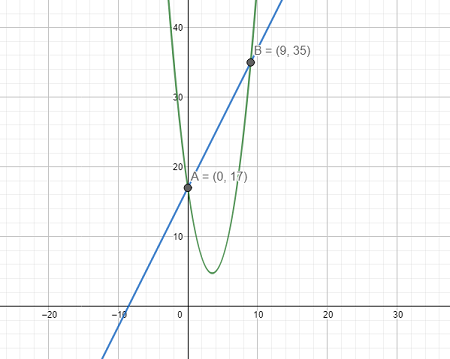# Find the area of the region bounded by y = x^2 - 7x + 17 and y = 2x + 17.

## Question:

Find the area of the region bounded by {eq}y = x^2 - 7x + 17 {/eq} and {eq}y = 2x + 17. {/eq}

## Area of Bounded Curves:

• When two curves are given by their graph it can be concluded whether they contain any common area between them or not. If there exists a common area between the two given curves, then one can determine the area of the region bounded by these curves with the help of calculus, to be more specific with the help of definite integration. The limits of the definite integration are decided by the graph.
• To evaluate area one can consider either a vertical or horizontal stripe. Out of these two strips which one is to be considered for a particular question is decided by the functions of the question. In some cases, the use of vertical will be beneficial as it would make the integration simpler whereas in other cases use of horizontal strip makes solving of interaction easier.
• Reverse power rule is the rule used to integrate the algebraic function in the form of polynomials. It can be given by the following formula:

$$\int x^n \ dx = \dfrac {x^{n+1}}{n+1} +C$$

where 'C' is the constant of integration.

Given:

First curve, parabola: {eq}y = x^2 - 7x + 17 {/eq}

Second Curve, straight line: {eq}y = 2x + 17 {/eq}

{eq}\\ {/eq}Consider a vertical strip between the two curves:

Area of the region bounded,

{eq}A = \int_0^9 \left ( (2x + 17) - (x^2 - 7x + 17) \right ) \ dx \\ \Rightarrow A = \int_0^9 \left ( 2x + 17 - x^2 + 7x - 17 \right ) \ dx \\ \Rightarrow A = \int_0^9 \left ( 2x - x^2 + 7x \right ) \ dx \\ \Rightarrow A = \int_0^9 \left ( 9x - x^2 \right ) \ dx \\ {/eq}

Integrating the above integral:

{eq}A = \left [ 9 ( \dfrac {x^{1+1}}{1+1} ) - \dfrac {x^{2+1}}{2+1} \right ]^9_0 \\ \Rightarrow A = \left [ 9 ( \dfrac {x^{2}}{2} ) - \dfrac {x^{3}}{3} \right ]^9_0 \\ \Rightarrow A = \left [ \dfrac {9}{2} x^2 - \dfrac {x^3}{3} \right ]^9_0 \\ {/eq}

Applying the limits to the integral:

{eq}A = \left [ (\dfrac {9}{2} (9^2) - \dfrac {9^3}{3} ) - ( \dfrac {9}{2} (0^2) - \dfrac {0^3}{3} ) \right ] \\ \Rightarrow A = \left [ (\dfrac {9}{2} (81) - \dfrac {9^3}{3} ) - ( 0 ) \right ] \\ \Rightarrow A = \left [ \dfrac {729}{2} - 243 \right ] \\ \Rightarrow A = 121.5 \ \text{units} \\ {/eq}

Therefore, the area of the region bounded by the given curves is {eq}121.5 \ \text{units}. {/eq}